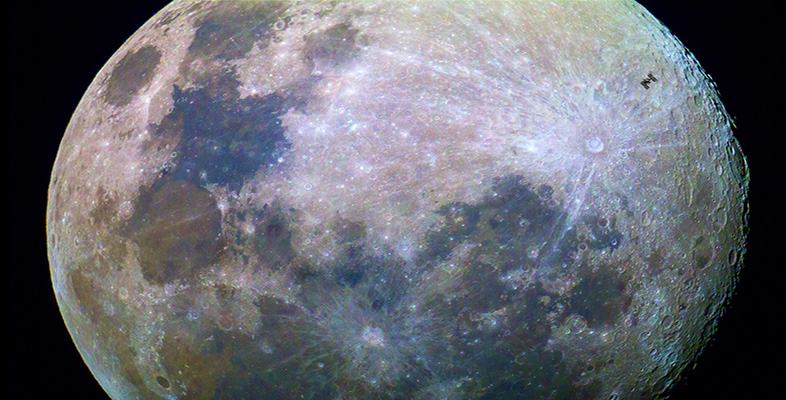Science, Maths & Technology

### Become an OU studentThe Moon

Start this free course now. Just create an account and sign in. Enrol and complete the course for a free statement of participation or digital badge if available.

# 3.14 Questions on the Moon

Now try to answer the following questions, to remind you of some of the things you have learned and test your understanding of them.

## Question 1

The first sentence of Section 2.4 describes the Moon's atmosphere as 'almost as insubstantial as Mercury's'. If Mercury's atmospheric pressure is 10-15 relative to that of the Earth, use the atmospheric pressure data in the Planetary facts table of Chapter 6 to calculate how the Moon's atmospheric pressure compares with Mercury's in terms of orders of magnitude. (Refer to Box 1 below if you need help.)

To use this interactive functionality a free OU account is required. Sign in or register.
Interactive feature not available in single page view (see it in standard view).

The Moon's atmospheric pressure is quoted as 2 × 10-14, relative to Earth, whereas Mercury's is 10-15. Thus, the Moon's atmospheric pressure relative to Mercury's is 2 × 10-14 / 10-15 = 2 × 101 = 20. This is closer to ten than to a hundred, so we would say that the Moon's atmospheric pressure is one order of magnitude greater than Mercury's.

## Box 1: Orders of magnitude and multiplying and dividing powers of ten

Orders of magnitude

Mercury's atmospheric pressure is quoted as 10-15 that of the Earth (we could write it more fully as '1 × 10-15', but the '1 ×' is redundant). In this case the power of ten is preceded by a minus sign, and it means that the number is 1 divided by 1 followed by 15 zeros (i.e. 1 divided by 1015, or 1/(1015), which is 0.000 000 000 000 001). That makes a very small number indeed. We can describe Mercury's atmospheric pressure as being 15 orders of magnitude less than the Earth's.

A value that is about ten times smaller than another is described as one order of magnitude smaller, about a hundred times smaller is described as two orders of magnitude smaller, etc. Turning this round, we can equally speak of one value being so many orders of magnitude greater than another. Values that differ by less than an order of magnitude are described as being 'of the same order of magnitude'. For example, 400 and 600 are of the same order of magnitude (as each other), and so are 9 × 103 and 2.5 × 104. Even though in the second case the two values have different powers of ten, the larger value is in fact less than three times greater than the smaller one.

Incidentally, you would say 10-15 as 'ten to the minus fifteen' and 1015 as 'ten to the fifteen'.

Multiplying and dividing powers of ten

When we multiply two numbers expressed in scientific notation, the rule is to multiply the numbers but add the powers of ten.

To calculate A × 10fmultiplied by B × 10g we simply work out A × B × 10f+g (note that we add the powers).

So, for example 3 × 106 × 5 × 103 = 3 × 5 × 106+3 = 15 × 108 (which we could express as 1.5 × 109 if we want to make the number before the × take a value of between 1 and 10).

On the other hand, to calculate A × 10fdivided by B × 10g we simply work out (A ÷ B) × 10f−g (note that we subtract the powers).

So, for example, 3 × 106 ÷ 5 × 103 = 3/5 × 106−3 = 0.6 × 103 (which we could express as 6 × 102 if we want to make the number before the × take a value of between 1 and 10).

## Question 2

Look at the basic properties of the Moon listed in the Planetary facts table of Chapter 6. Which of these do you think is likely to exert the greatest influence on the ease with which liquid lava can flow on the Moon relative to its behaviour on the Earth?

To use this interactive functionality a free OU account is required. Sign in or register.
Interactive feature not available in single page view (see it in standard view).

You might have picked temperature, on the grounds that lava will solidify quicker on a colder body. However, the Moon's average surface temperature is not much lower than the Earth's, so this is not actually the principal factor. The big difference between the two bodies is surface gravity. The Moon's surface gravity relative to the Earth is only 0.17 (about a sixth of the Earth's). This means that the force acting to spread flowing lava away from its source is six times weaker on the Moon than on the Earth. Lunar lava flows should thus tend to be thicker than equivalent flows on Earth. [Comment: This may be one of the reasons why lava tubes on the Moon have been able to attain much bigger dimensions than lava tubes on the Earth.]

## Question 3

Which statement about the Moon in the list is correct?

a.

A. Apollo seismic data show that the Moon's lithosphere is on average about 70 km thick.

b.

B. The Moon's core is thought to be at least 1290 km across.

c.

C. The lunar highlands are older than the maria.

d.

D. There are no lava flows on the Moon.

e.

E. The outer part of the core is liquid.